How much is 90 degrees Celsius in Fahrenheit?Denisse

Concept:

Temperature can be expressed in three different units namely,

1. Degree Celsius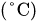2. Kelvin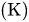3. Fahrenheit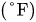Step: 1 The S.I unit of temperature is Kelvin. One unit can be converted into another using the proper conversion factor.

Step: 2  The conversion factor to convert temperature from degree Celsius to Kelvin is,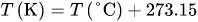...... (1)

Step: 3  The conversion factor to convert temperature from degree Celsius to Fahrenheit is,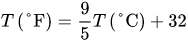...... (2)

Solution:

Step: 1 The given temperature value is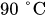.

Step: 2 Substitutefor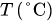in equation (2) to calculate the temperature in Fahrenheit scale.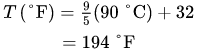Therefore, 90 degrees Celsius in Fahrenheit is.

Common mistake:

Use correct formula to calculate the temperature in Fahrenheit scale.

Similar problems:

Question:

1. How much is 22 degrees Celsius in Fahrenheit?

Solution:

Step: 1 The given temperature value is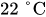.

Step: 2 Substituteforin equation (2) to calculate the temperature in Fahrenheit scale.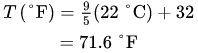Therefore, 22 degrees Celsius in Fahrenheit is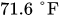.

Common mistake:

Use correct formula to calculate the temperature in Fahrenheit scale.

Question:

2. How much is 34 degrees Celsius in Fahrenheit?

Solution:

Step: 1 The given temperature value is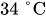.

Step: 2 Substituteforin equation (2) to calculate the temperature in Fahrenheit scale.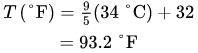Therefore, 34 degrees Celsius in Fahrenheit is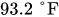.

Common mistake:

Use correct formula to calculate the temperature in Fahrenheit scale.

Need homework help now?

tutors online

Related Questions
2. (11 marks) a) At 400 °C, ammonium iodide decomposes in the following endothermic reaction: NH41(s) + NH3(g) + HI(g) Kp = 0.215 at 400 °C. Write the equilibrium expression for this reaction. 15.0 g of NH4I was placed in an empty 3.0 L flask and heated to 400 °C. What is the partial pressure of NH3 in the flask once the reaction has reached equilibrium? How would the following changes to the equilibrium mixture affect the number of moles of NH3 in the flask and the value of K, once equilibrium is re-established? (Use increase, decrease or stay the same.) Change Effect on moles of NH3 Ke value Increase temperature Increase volume of flask Remove half of HI gas
Notes
Me

OR

Don't have an account?

Join OneClass

Access over 10 million pages of study
documents for 1.3 million courses.

Join to view

OR

By registering, I agree to the Terms and Privacy Policies
Just a few more details

So we can recommend you notes for your school.